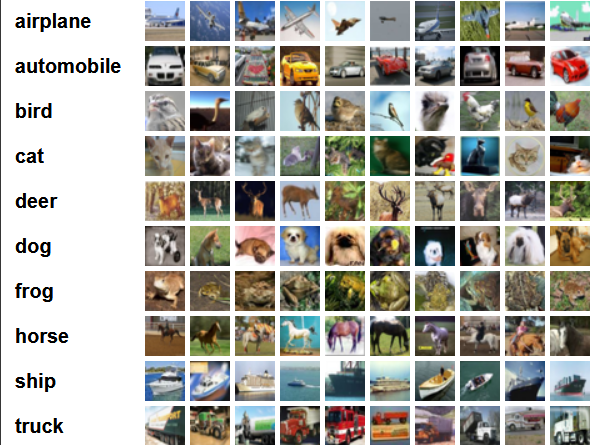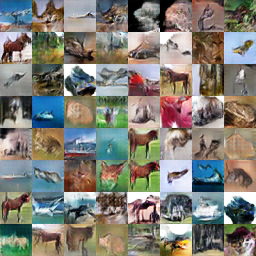cifar10数据集是比较难训练和分类识别的，对于GAN模型生成cifar10数据对其特性也是一个不小的挑战。如果GAN模型可以很好的生成 cifar10数据图片的话，这个GAN的生成能力已经是很不错的了。今天我们说说如何把下载下来的cifar10数据集输出到GAN模型中用作训练。def load_cifar10(dataset_name):
data_dir = os.path.join("./data", dataset_name)

X_train = []
Y_train = []

dirname = './data/cifar/cifar-10-batches-py'

for i in range(1, 6):
fpath = os.path.join(dirname, 'data_batch_' + str(i))
if i == 1:
X_train = data
Y_train = labels
else:
X_train = np.concatenate([X_train, data], axis=0)
Y_train = np.concatenate([Y_train, labels], axis=0)

fpath = os.path.join(dirname, 'test_batch')

X_train = np.dstack((X_train[:, :1024], X_train[:, 1024:2048],
X_train[:, 2048:])) / 255.
X_train = np.reshape(X_train, [-1, 32, 32, 3])
X_test = np.dstack((X_test[:, :1024], X_test[:, 1024:2048],
X_test[:, 2048:])) / 255.
X_test = np.reshape(X_test, [-1, 32, 32, 3])

X = np.concatenate((X_train, X_test), axis=0)

# if one_hot:
#    Y_train = to_categorical(Y_train, 10)
#    Y_test = to_categorical(Y_test, 10)

Y_test = np.asarray(Y_test)
y = np.concatenate((Y_train, Y_test), axis=0).astype(np.int)

seed = 547
np.random.seed(seed) #确保每次生成的随机数相同
np.random.shuffle(X) #将mnist数据集中数据的位置打乱
np.random.seed(seed)
np.random.shuffle(y)

y_vec = np.zeros((len(y), 10), dtype=np.float)
#创建了(70000,10)的标签记录，并且根据cifar10已有标签记录相应的10维数组
for i, label in enumerate(y):
y_vec[i, y[i]] = 1.0

#返回归一化的数据和标签数组
return X, y_veccifar10的生成效果还是一般般的，原因也是在于cifar10难于训练的，期待更好的模型完成图像生成。